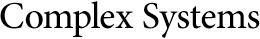## A Symbolic Way to Express Paths and Orbits of Two Iterated Function Systems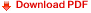Horacio A. Caruso
Two iterated function systems, resulting in the attractors generally known as the Sierpinski triangle and the von Koch snow flake curve, are studied. The complete attractor is considered to be at the level of precision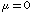; it is divided into smaller replicas (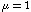), each of which in turn are considered to be composed of smaller replicas (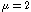), and so on up to higher levels of precision. An algorithm is designed to represent paths at any (finite) level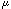and a very simple procedure is found to simulate random orbits. From these orbits periods may be found. A statistical study of periods shows that, as the level of precision tends to infinity, the mean period also tends to infinity